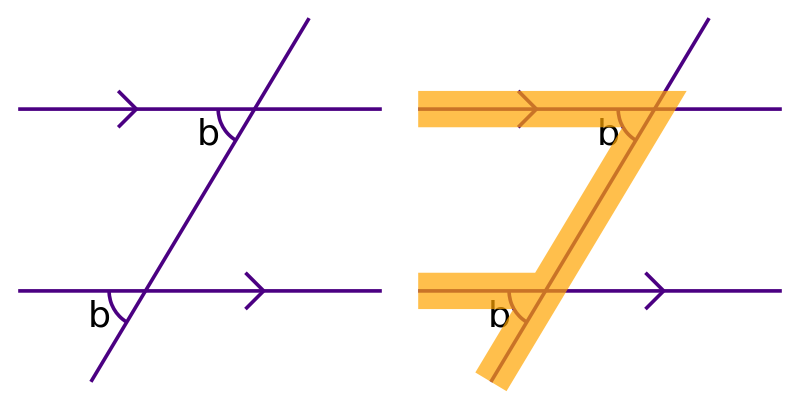# Parallel lines

Martin McBride
2020-08-15

Here are some basic geometry rules about parallel lines. They are also covered in this video:

## Angles around intersection lines

When two lines intersect, four angles are a formed: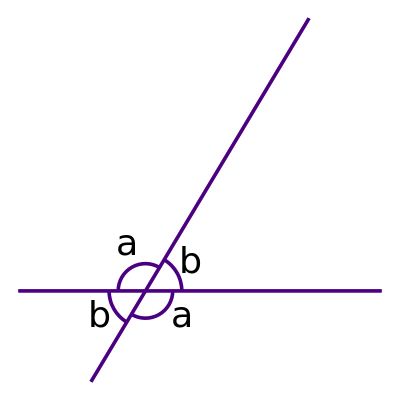The angles around intersecting lines form two pairs of opposite and equal angles. The two angles labelled a are equal, and the two angles labelled b are equal. This means that there are only two different angles at the intersection.

Since angles a and b form a straight line, we also know that:

a + b = 180°


## Angles around parallel lines

If we draw an extra line, parallel to the previous line, it looks like this: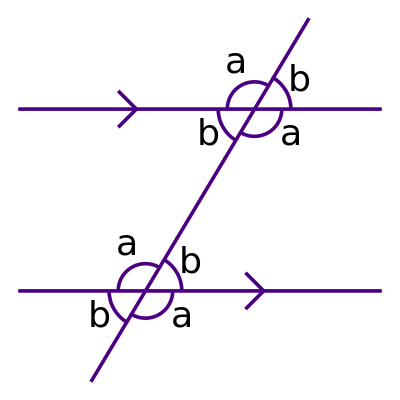The small arrows on the two horizontal lines indicate that they are parallel with each other.

Since the lines are parallel, they both make the same angle with the line that crosses them. So both intersections only involve the two angles a and b. This means that if we know the value of any angle, we can find out all the other angles. For example, if we are told that a is 120°, we know that all the angles labelled a in the diagram are 120°. We can then calculate that b is 60° (since a + b is 180°), so we know all the angles.

There are several common cases of matching pairs of angles around pairs of angles. These are given special names - alternate angles, allied angles, and corresponding angles.

## Alternate angles

If you take one of the angles from the first intersection, and the opposite angle from the second intersection, they are called alternate angles: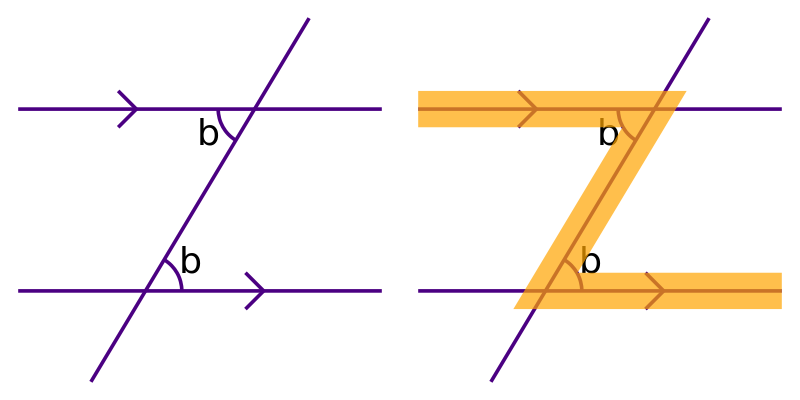Alternate angles are equal. In this case the angles are both equal to b.

The diagram shows that you can spot alternate angles by a characteristic Z shape. But the shape might be rotated or flipped so it might not always look exactly like a Z. Here is an example of a different pair of alternate angles: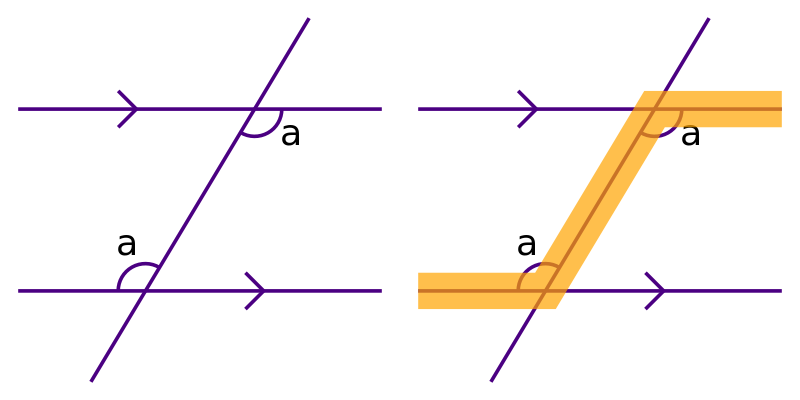## Allied angles

You could think of the intersection as being one half of a parallelogram: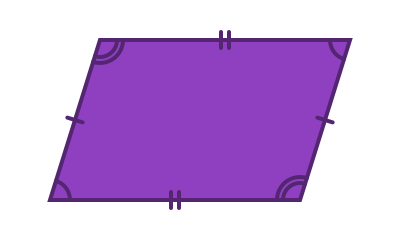Allied angles are the two "internal" angles in that half of the parallelogram: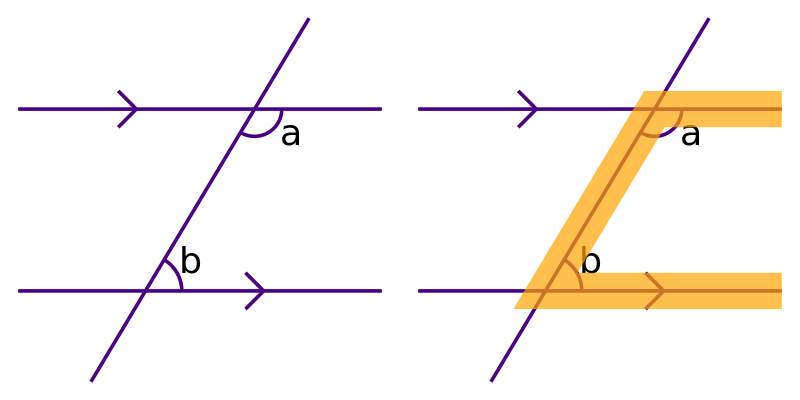Allied angles add up to 180°. We can see this because there will always be one a and one b angle.

Allied angles are sometimes called co-linear angles or internal angles.

The diagram shows that you can spot allied angles by a characteristic C shape. The shape might be rotated or flipped so it might not always look exactly like a C. Here is an example of a different pair of allied angles: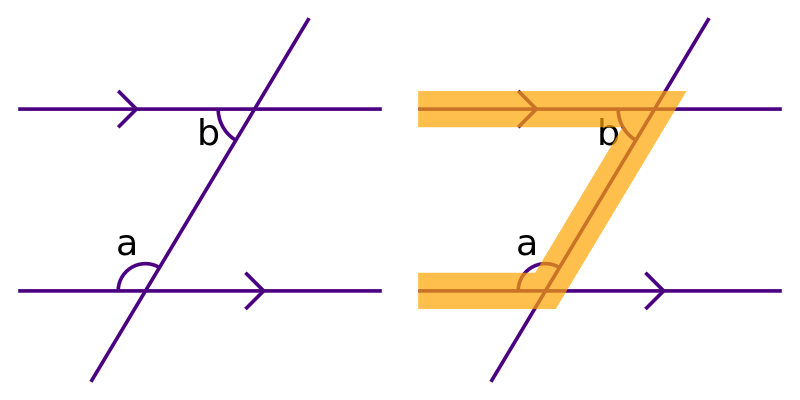## Corresponding angles

If you take one of the angles from the first intersection, and the equivalent angle from the second intersection, they are called corresponding angles: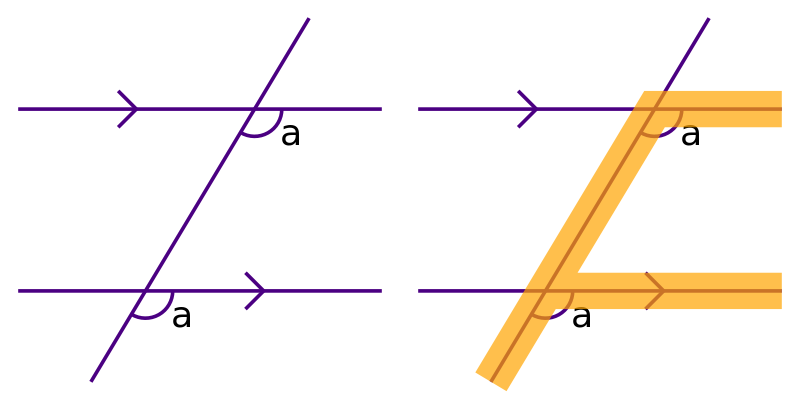Corresponding angles are equal. In this case the angles are both equal to a.

The diagram shows that you can spot corresponding angles by a characteristic F shape. Again, the shape might be rotated or flipped so it might not always look exactly like an F. Here is an example of a different pair of corresponding angles: